RS Aggarwal Test: Pair of Linear Equations in Two Variables

# RS Aggarwal Test: Pair of Linear Equations in Two Variables - Class 10

Test Description

## 10 Questions MCQ Test Mathematics (Maths) Class 10 - RS Aggarwal Test: Pair of Linear Equations in Two Variables

RS Aggarwal Test: Pair of Linear Equations in Two Variables for Class 10 2023 is part of Mathematics (Maths) Class 10 preparation. The RS Aggarwal Test: Pair of Linear Equations in Two Variables questions and answers have been prepared according to the Class 10 exam syllabus.The RS Aggarwal Test: Pair of Linear Equations in Two Variables MCQs are made for Class 10 2023 Exam. Find important definitions, questions, notes, meanings, examples, exercises, MCQs and online tests for RS Aggarwal Test: Pair of Linear Equations in Two Variables below.
Solutions of RS Aggarwal Test: Pair of Linear Equations in Two Variables questions in English are available as part of our Mathematics (Maths) Class 10 for Class 10 & RS Aggarwal Test: Pair of Linear Equations in Two Variables solutions in Hindi for Mathematics (Maths) Class 10 course. Download more important topics, notes, lectures and mock test series for Class 10 Exam by signing up for free. Attempt RS Aggarwal Test: Pair of Linear Equations in Two Variables | 10 questions in 10 minutes | Mock test for Class 10 preparation | Free important questions MCQ to study Mathematics (Maths) Class 10 for Class 10 Exam | Download free PDF with solutions
 1 Crore+ students have signed up on EduRev. Have you?
RS Aggarwal Test: Pair of Linear Equations in Two Variables - Question 1

### If the lines given by 3 x + 2 ky = 2 and 2x + 5y + 1 = 0 are parallel, then value of k is

Detailed Solution for RS Aggarwal Test: Pair of Linear Equations in Two Variables - Question 1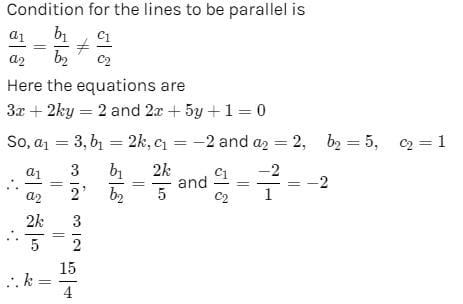RS Aggarwal Test: Pair of Linear Equations in Two Variables - Question 2

### The pair of equations ax + 2y = 7 and 3x + by = 16 represent parallel lines if

Detailed Solution for RS Aggarwal Test: Pair of Linear Equations in Two Variables - Question 2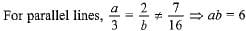RS Aggarwal Test: Pair of Linear Equations in Two Variables - Question 3

### If x = a, y = b is the solution of the equations x - y = 2 and x + y = 4, then the values of a and b are, respectively

RS Aggarwal Test: Pair of Linear Equations in Two Variables - Question 4

The equations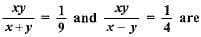equivalent to the equations:

Detailed Solution for RS Aggarwal Test: Pair of Linear Equations in Two Variables - Question 4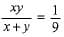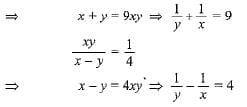RS Aggarwal Test: Pair of Linear Equations in Two Variables - Question 5

The pair of equations 5x - 15y = 8 and 3x - 9y = 24/5 has

RS Aggarwal Test: Pair of Linear Equations in Two Variables - Question 6

The value of k for which the system of equations 2x + ky = 12, x + 3y - 4 = 0 are inconsistent is

RS Aggarwal Test: Pair of Linear Equations in Two Variables - Question 7

The pair of equations y = 0 and y = -7 has

RS Aggarwal Test: Pair of Linear Equations in Two Variables - Question 8

Graphically, the pair of equations 6x - 3y + 10 = 0; 2x - y + 9 = 0 represents two lines which are

RS Aggarwal Test: Pair of Linear Equations in Two Variables - Question 9

Five years ago, A was thrice as old as B and ten years later, A shall be twice as old as B. What is the present age of A?

RS Aggarwal Test: Pair of Linear Equations in Two Variables - Question 10

One equation of a pair of dependent linear equations is - 5x +7y = 2. The second equation can be

## Mathematics (Maths) Class 10

115 videos|478 docs|129 tests
Information about RS Aggarwal Test: Pair of Linear Equations in Two Variables Page
In this test you can find the Exam questions for RS Aggarwal Test: Pair of Linear Equations in Two Variables solved & explained in the simplest way possible. Besides giving Questions and answers for RS Aggarwal Test: Pair of Linear Equations in Two Variables, EduRev gives you an ample number of Online tests for practice

## Mathematics (Maths) Class 10

115 videos|478 docs|129 tests